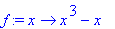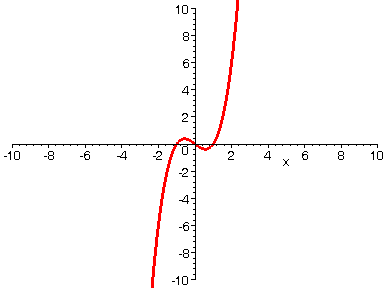HORIZONTAL SHIFT

If you take a function y = f(x) and replace it by y = f(x - k) where k > 0, then the result is that the graph of f(x) is shifted horizontally to the right. In a similar manner, y = f(x + k) would produce a horizontal shift to the left. Below we start with a polynomial function of the form y = f(x - k), and the animation shows the effect on the graph as we let k increase from 0 to 10.

> with(plots):

> f:=x->x^3-1*x;> A := seq(plot(f(x - i), x=-10..10, numpoints=500, thickness=3), i=0..10):

> display(A, insequence=true, view=[-10..10, -10..10]);>